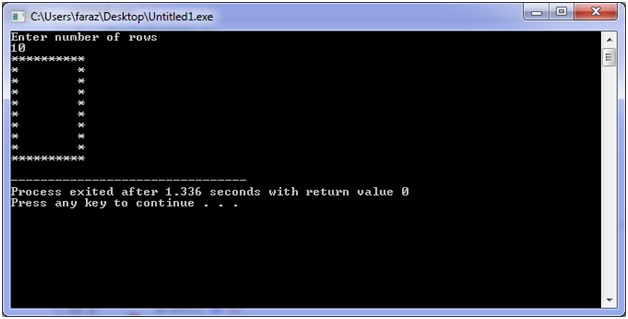# C program for 'Hollow Square' Pattern

In this C program, we are going to create, print a Hollow Square using asterisks and loops (nested loops).
Submitted by Shamikh Faraz, on February 16, 2018

In this program we will learn, how we can print hollow square using ‘asterisks’ and 'loops'?

C program

﻿

```#include<stdio.h>

int main(){
int rows, a, b;
printf("Enter number of rows\n");
scanf("%d", &rows);

/*this loop increase number of rows */
for(a = 0; a < rows; a++)
{
/*this loop increase number of columns */
for(b = 0; b < rows; b++)
{
/* this puts the star on boundaries */
if(a==0 || a==rows-1 || b==0 || b==rows-1)
printf("*"); //prints the star
else
printf(" "); /* leaves the middle columns of square blank*/
}
printf("\n");
}
return 0;
}
```

Output